Register for Maths, Science, English, Reasoning Olympiad Exams Register here | Book Free Demo for Live Olympiad Classes here | Check Olympiad Exam Dates here | Buy Practice Papers for IMO, IOM, HEO, IOEL etc here | Login here to participate in all India free mock test on every Saturday

# Introduction to Graphs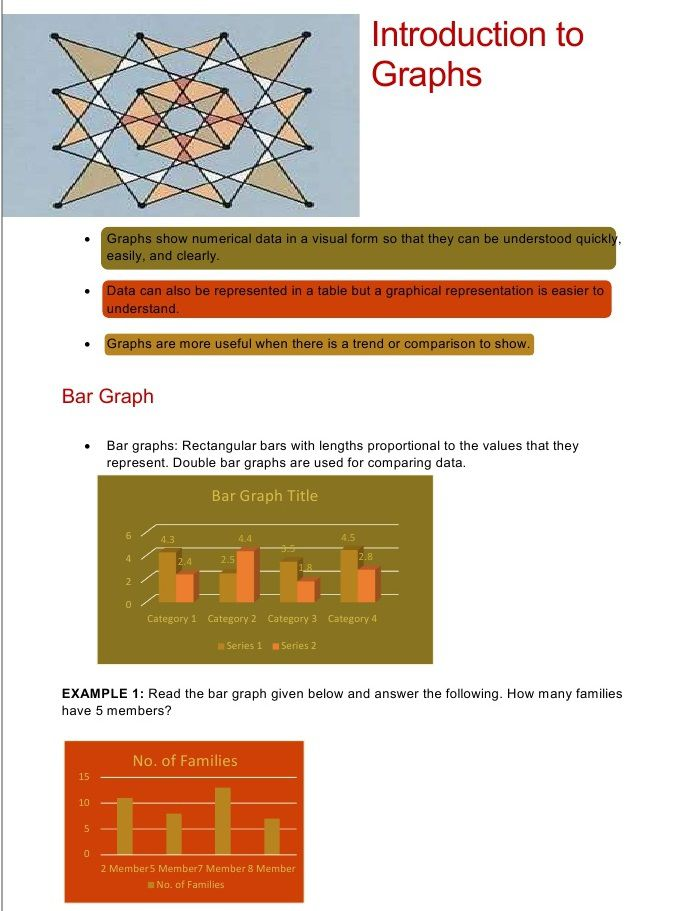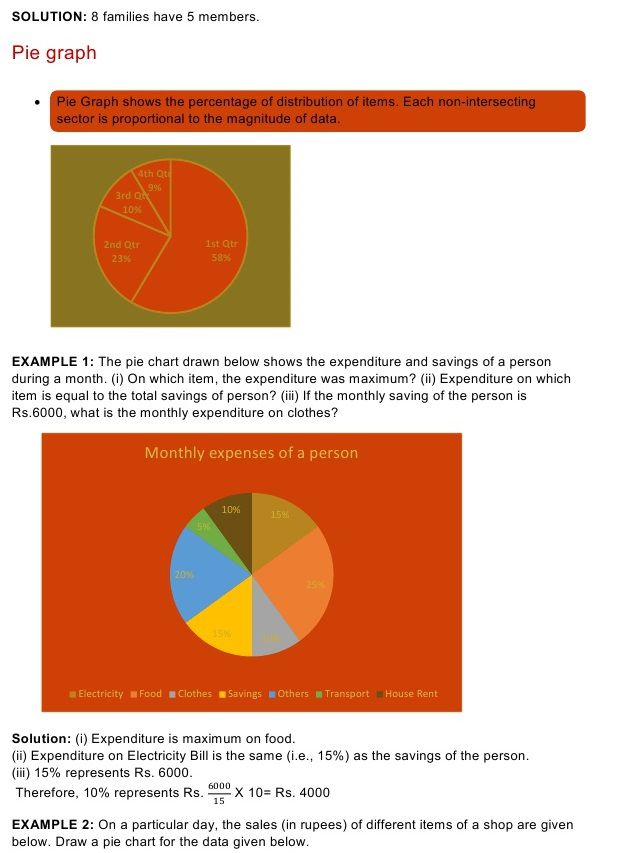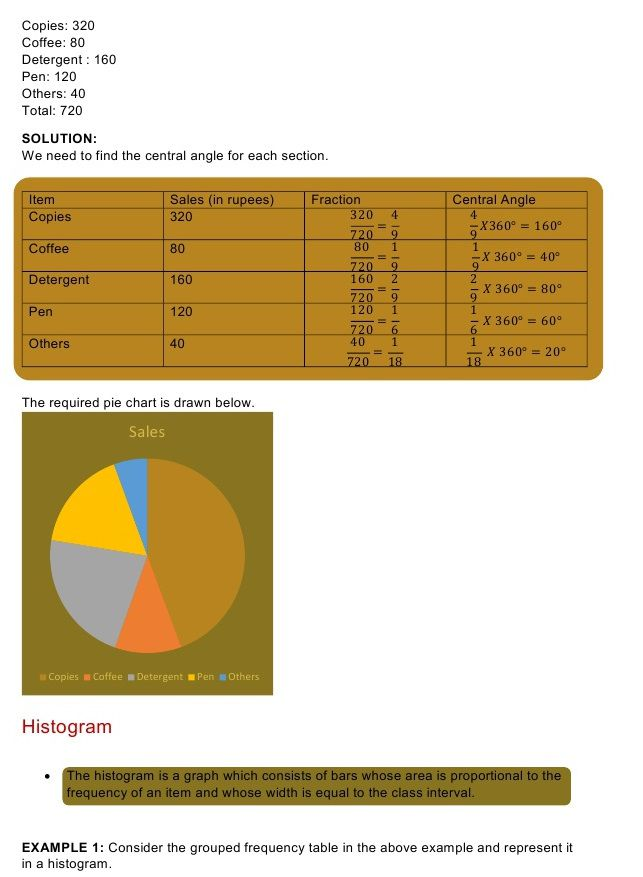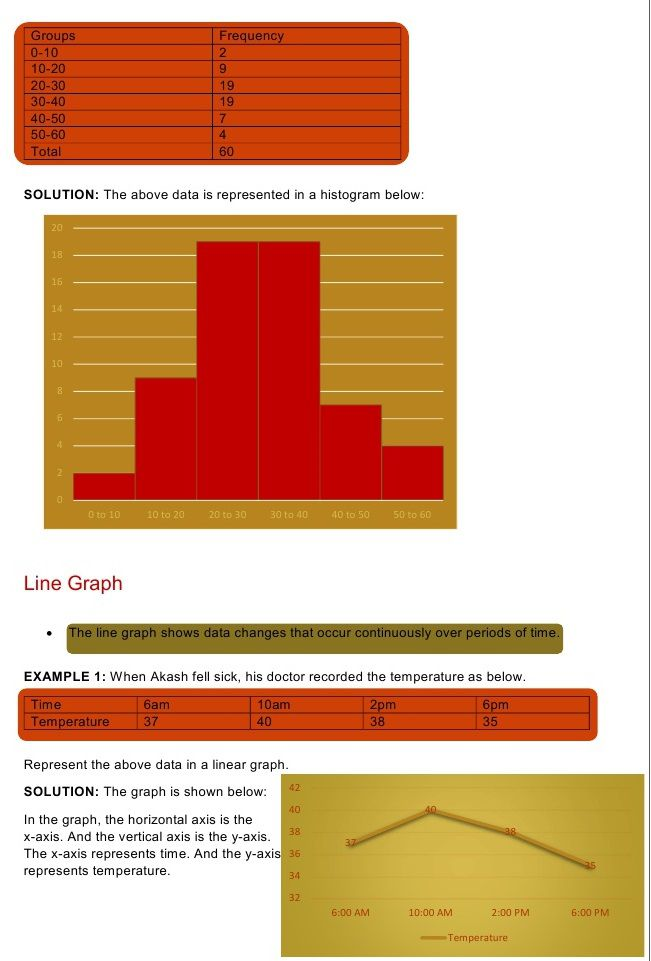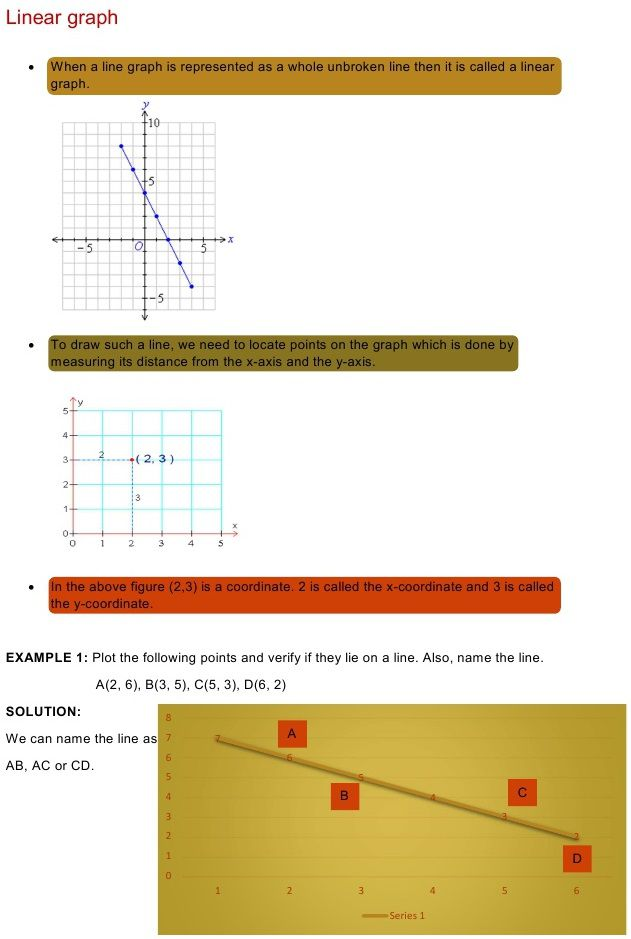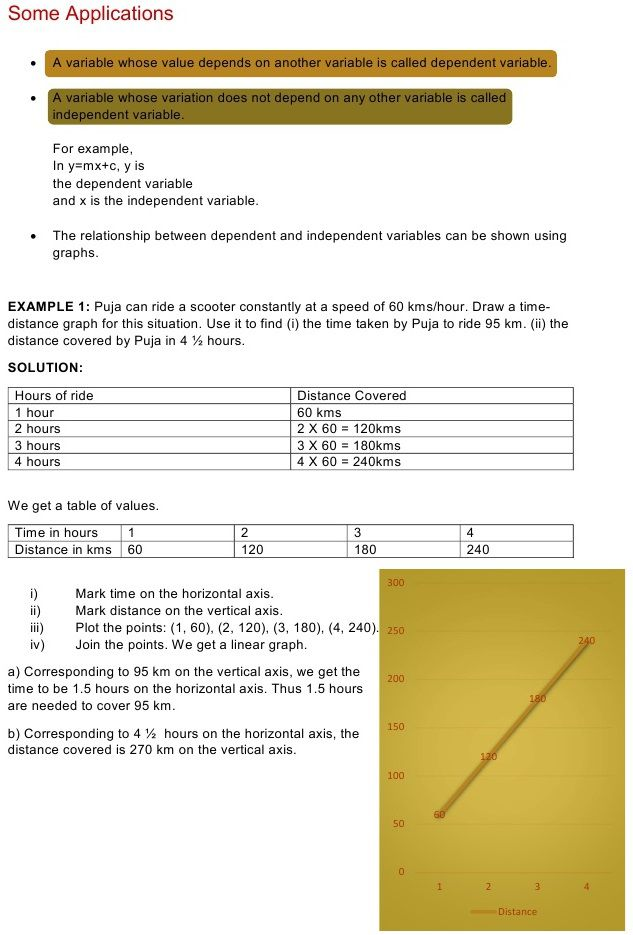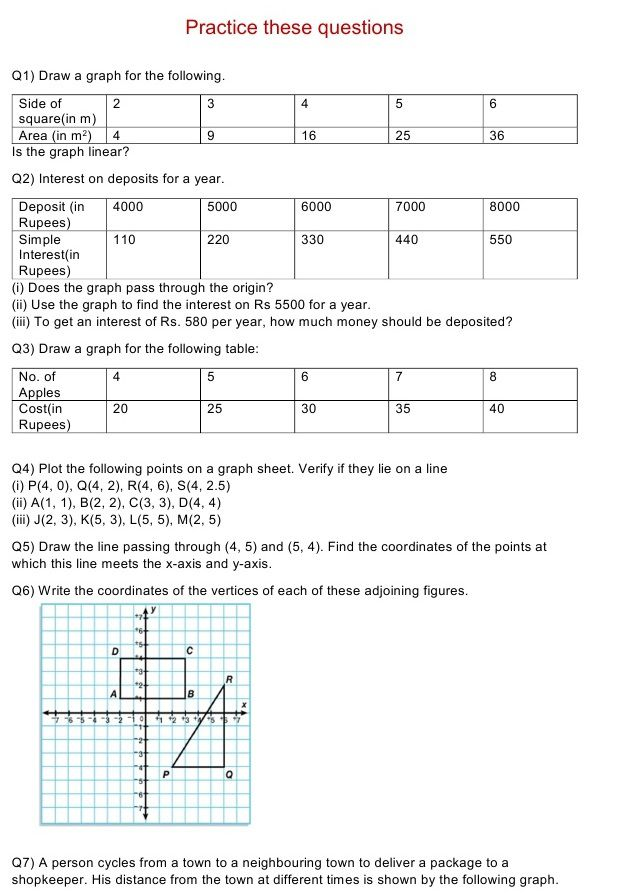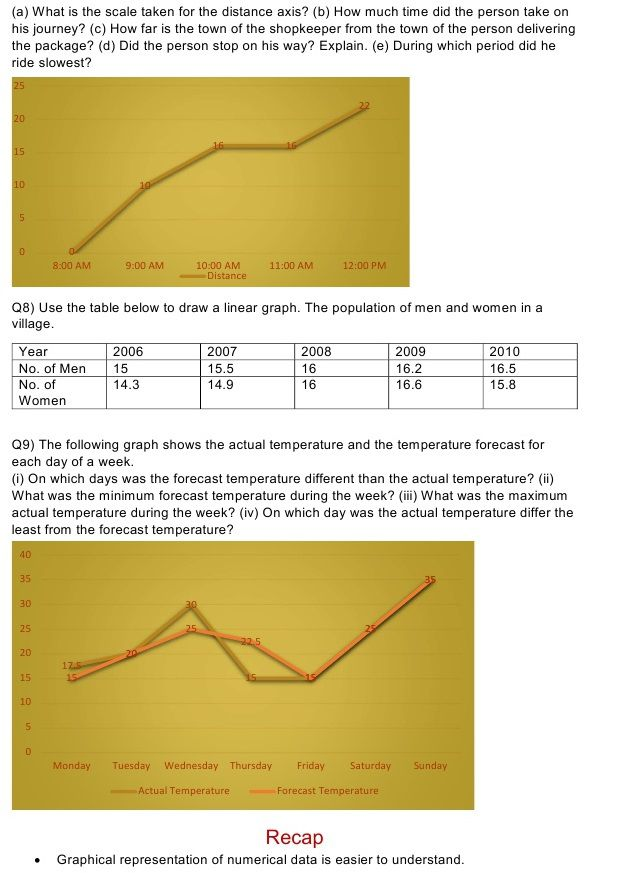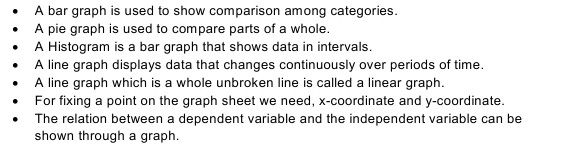## Quiz for Introduction to Graphs

 Q.1 Which point represents the origin? a) (1, 1) b) (0, 0) c) (-2, 2) d) (0, 1)
 Q.2 The point (8, 0) will lie where? a) 1st quadrant b) 2nd quadrant c) On y-axis d) On x-axis
 Q.5 Find the coordinates that represent the point B.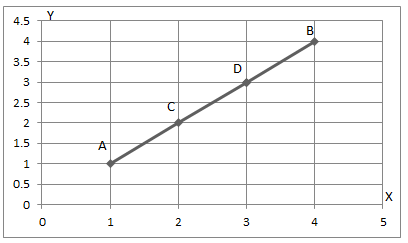a) (1, 1) b) (4, 4) c) (2, 2) d) (3, 3)
 Q.6 Find the coordinates that represent the point B.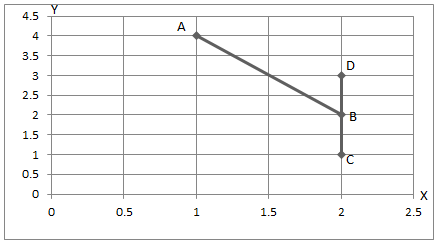a) (1, 4) b) (2, 3) c) (3, 4) d) (2, 2)
 Q.7 Find the coordinates that represent the point E.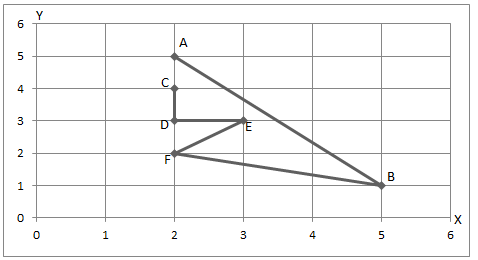a) (2, 5) b) (3, 4) c) (3, 3) d) (2, 2)
 Q.8 Find the coordinates of point C.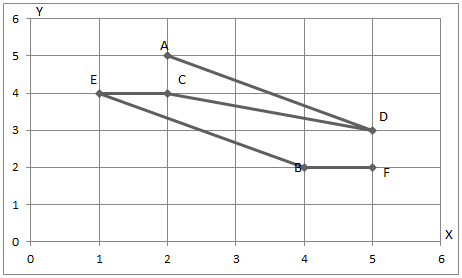a) (2, 4) b) (2, 5) c) (1, 4) d) (5, 3)
 Q.9 Find the coordinates of point A.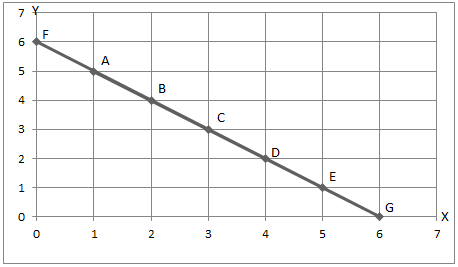a) (0, 6) b) (2, 4) c) (1, 5) d) (5, 1)
 Q.10 If there are total 600 students in a school and the distribution of students in each class is represented in the given pie-chart, find how many students are there in class 6?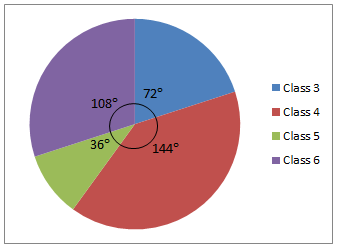a) 60 b) 240 c) 120 d) 108